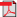# How To Answer a Problem Question

This book explains how to answers a problem question. It does this by providing a model. Naturally this model incorporates advice on how to perform the four fundamental skills that are needed to answer a problem question.
Sales price \$45.00
Sales price without tax \$40.91
Tax amount \$4.09
Description

by Christopher Enright

Problem questions are a standard exercise for teaching law students about the law and for training the students in some important skills for working with law. For these reasons problem questions are also a common type of question for assessing law students in exams and assignments. Problem questions also help law students in their practical training because they imitate the task that lawyers perform when they advise a client, typically in circumstances where there is a possibility of litigation.
Answering a problem question requires four fundamental legal skills that lawyers need. These are the skills of organising law, applying law to facts, interpreting law and writing law.

This book explains how to answers a problem question. It does this by providing a model. Naturally this model incorporates advice on how to perform the four fundamental skills that are needed to answer a problem question.
Chapter 1 sets out the model for answering a problem question in a diagram. The diagram takes the following form:

• Step 1.  Performing Tasks for the Facts
• Step 2.  Constructing a Framework
• Step 3.  Applying Law
• Step 4.  Interpreting Law
• 4.1  Identify the Meanings and Effects
• 4.2  Identify the Purpose and Object
• 4.3  Identify the Correct Meaning
• Step 5.  Determining the Outcome
• Step 6.  Writing the Answer

The book has three parts:

• Chapter 1 explains the nature and use of problem questions.
• Chapters 2-7 explain in turn each of the steps in answering a problem question.
• Chapter 8 illustrates the task of answering a problem question. It sets up the illustration by presenting a problem question that is based on a set of hypothetical facts and a provision in a hypothetical statute. It then states the task to be done which is to advise one of the parties. The ensuing illustration proceeds through each of the six steps in turn. It performs each step and where appropriate gives advice. In concludes by presenting a version of the answer. Needless to say there is often room to argue as to what should or should not be in an advice or there can be different ways of saying the same thing. Consequently the answer that Chapter 8 presents is not holy writ, merely an illustration.

124 pages softcoverView a sample of this book.